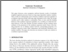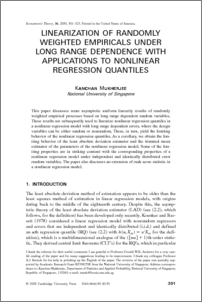Linearization of randomly weighted empiricals under long range dependence with application to nonlinear regression quantiles

Mukherjee, Kanchan (2000) Linearization of randomly weighted empiricals under long range dependence with application to nonlinear regression quantiles. Econometric Theory, 16 (3). pp. 301-323. ISSN 1469-4360Preview
PDF
S0266466600163017a.pdf - Published Version

Abstract

This paper discusses some asymptotic uniform linearity results of randomly weighted empirical processes based on long range dependent random variables+ These results are subsequently used to linearize nonlinear regression quantiles in a nonlinear regression model with long range dependent errors, where the design variables can be either random or nonrandom+ These, in turn, yield the limiting behavior of the nonlinear regression quantiles+ As a corollary, we obtain the limiting behavior of the least absolute deviation estimator and the trimmed mean estimator of the parameters of the nonlinear regression model+ Some of the limiting properties are in striking contrast with the corresponding properties of a nonlinear regression model under independent and identically distributed error random variables+ The paper also discusses an extension of rank score statistic in a nonlinear regression model+

Item Type:
Journal Article
Journal or Publication Title:
Econometric Theory
http://journals.cambridge.org/action/displayJournal?jid=ECT The final, definitive version of this article has been published in the Journal, Econometric Theory, 16 (3), pp 301-323 2000, © 2000 Cambridge University
Uncontrolled Keywords:
/dk/atira/pure/subjectarea/asjc/3300/3301
Subjects:
Departments:
ID Code:
65676
Deposited By:
Deposited On:
15 Jul 2013 09:11
Refereed?:
Yes
Published?:
Published